# Exclusive NOR gate

Table of Content:

Exclusive-NOR gate or XNOR gate is formed by combining the Exclusive-OR gate (XOR gate) and the NOT gate. On analysing the XNOR truth table, we understand that the output is similar to the standard NOR gate except that the output of the XNOR gate is high when both inputs are high. The output of EX-NOR gate is 1 when both the inputs are 1 (high) and if both the inputs are 0 (low). If either one of the input is 1 (high) the output will be 0 (low). In other words, both the inputs should be in the same logic level for the output to be 1.

## Symbol and Boolean expression for XNOR gate

A simple NOR gate symbol can be denoted by a standard OR gate with an inversion bubble connected. The logic symbol of an Exclusive-NOR gate is an XOR gate (Exclusive-OR gate) with the “inversion bubble” or the circle in front. Therefore, the Exclusive-NOR gate is the complementary form of the Exclusive-OR gate.

The Boolean expression for the XNOR gate is given by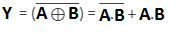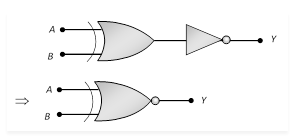## XNOR Gate Truth Table for 2-input

 A B Y 0 0 1 0 1 0 1 0 0 1 1 1

The same logic can be used to find the output of 3 input Ex-NOR gate. The Boolean expression for the same is given by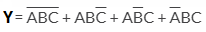. The truth table for 3 input is given below

## XNOR Gate Truth Table for 3-input

 A B C Y 0 0 0 1 0 0 1 0 0 1 0 0 0 1 1 1 1 0 0 0 1 0 1 1 1 1 0 1 1 1 1 0

## Exclusive-NOR gate equivalent circuit

The Ex-NOR gate can be constructed by combining the OR gate, AND gate and NOT gate.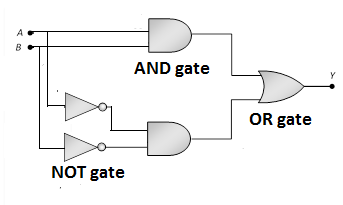One easier way of constructing the XNOR gate is from using a single gate like the AND gate.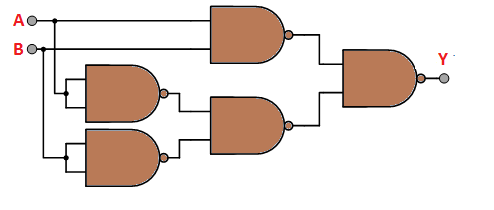## Application of Exclusive-NOR gate

Some of the application of XNOR gate is in

• Encryption and arithmetic circuits
• Error-detecting circuits to find odd parity and even parity in digital data transmission.

### Recommended Video:

Current Electricity Class 12 Physics One Shot & Mind Maps | Physics Ch 3 Class 12 | NEET 2022 Exam